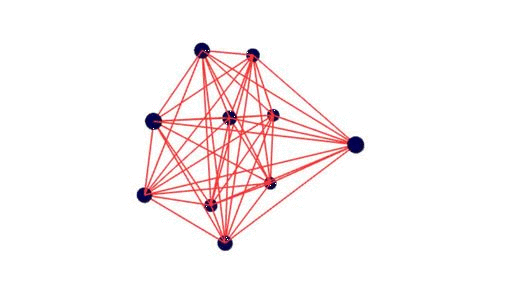# Plotting Graphs

LightGraphs.jl integrates with several other Julia packages for plotting. Here are a few examples.

## GraphLayout.jl

This excellent graph visualization package can be used with LightGraphs.jl as follows:

julia> g = wheel_graph(10); am = Matrix(adjacency_matrix(g))
julia> loc_x, loc_y = layout_spring_adj(am)
julia> draw_layout_adj(am, loc_x, loc_y, filename="wheel10.svg")

producing a graph like this:## TikzGraphs.jl

Another nice graph visualization package. (TikzPictures.jl required to render/save):

julia> g = wheel_graph(10); t = plot(g)

julia> save(SVG("wheel10.svg"), t)

producing a graph like this:## GraphPlot.jl

Another graph visualization package that is very simple to use. Compose.jl is required for most rendering functionality:

julia> using GraphPlot, Compose

julia> g = wheel_graph(10)

julia> draw(PNG("/tmp/wheel10.png", 16cm, 16cm), gplot(g))

## NetworkViz.jl

NetworkViz.jl is tightly coupled with LightGraphs.jl. Graphs can be visualized in 2D as well as 3D using ThreeJS.jl and Escher.jl.

#Run this code in Escher

using NetworkViz
using LightGraphs

main(window) = begin
push!(window.assets, "widgets")
push!(window.assets,("ThreeJS","threejs"))
g = complete_graph(10)
drawGraph(g)
end

The above code produces the following output:## SGtSNEpi.jl

SGtSNEpi.jl is a high-performance software for swift embedding of a large, sparse graph into a d-dimensional space (d = 1,2,3). The Makie plotting ecosystem is used for interactive plots.

using GLMakie, SGtSNEpi, SNAPDatasets

GLMakie.activate!()

g = loadsnap(:as_caida)
y = sgtsnepi(g);
show_embedding(y;
A = adjacency_matrix(g),        # show edges on embedding
mrk_size = 1,                   # control node sizes
lwd_in = 0.01, lwd_out = 0.001, # control edge widths
edge_alpha = 0.03 )             # control edge transparency

The above code produces the following output:SGtSNEpi.jl enables 3D graph embedding as well. The 3D embedding of the weighted undirected graph ML_Graph/optdigits_10NN is shown below. It consists of 26,475 nodes and 53,381 edges. Nodes are colored according to labels provided with the dataset.